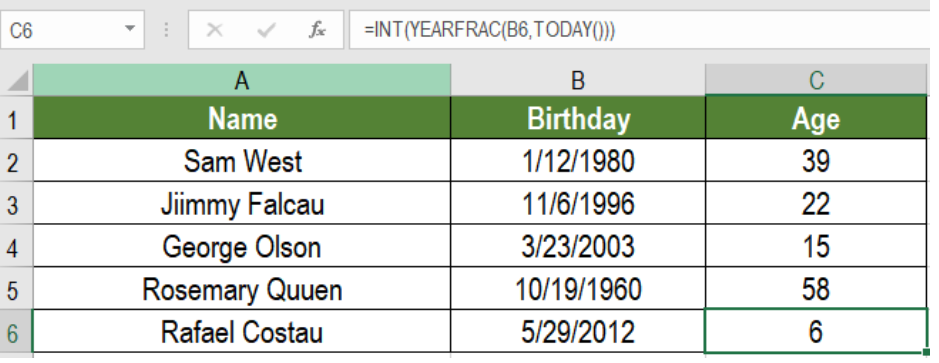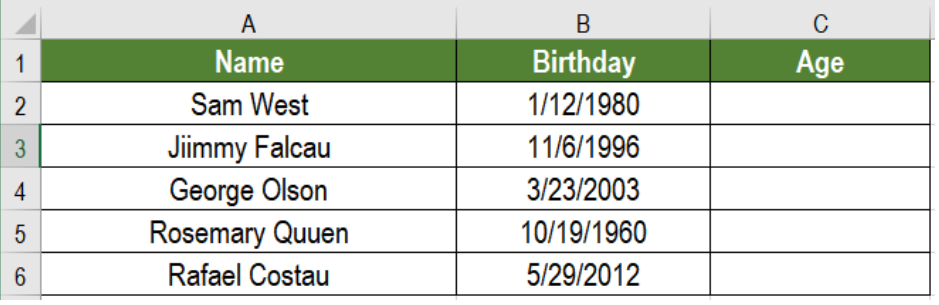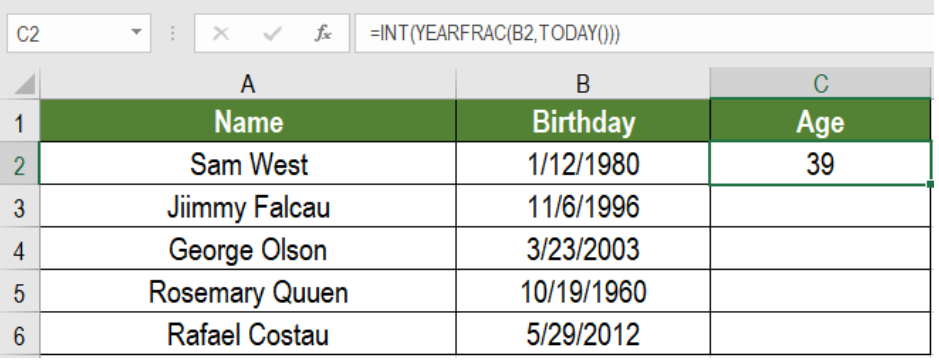Get instant live expert help with Excel or Google Sheets“My Excelchat expert helped me in less than 20 minutes, saving me what would have been 5 hours of work!”

#### Post your problem and you’ll get expert help in seconds.

Your message must be at least 40 characters
Our professional experts are available now. Your privacy is guaranteed.

# Get age from birthday

We often need to find the age of an individual for different purposes. It is often required for official and personal purposes. The calculations to calculate age can be tricky. However, Excel allows us to get age from birthday very easily. We can use the INT, YEARFRAC and TODAY function in Excel to calculate age from birthday. In this tutorial, we will learn how to get age from birthday in Excel.Figure 1. Example of How to Get Age from Birthday

## General Formula

`=INT(YEARFRAC(birth_date,TODAY()))`

## How this Formula Works

Here, you just need to provide the date of birth inside the YEARFRAC function. The second argument is the TODAY function. This also ensures the age is correct every time the spreadsheet is opened.

YEARFRAC takes two dates and returns a decimal number. This represents the fraction of a year between them. Excel uses full days between two dates to calculate the fraction of a year as a decimal. As all dates are serial numbers, this is the default for Excel. Afterwards, the INT function rounds this fraction number to an integer,and returns it.

## Setting Up Data

The following uses a sample people information database. Column A and B has the name and birthdates of some people.Figure 2. The Sample Data

To calculate the ages from the birthdates, we need to

• Go to cell C2.
• Assign the formula `=INT(YEARFRAC(B2,TODAY()))` to C2.
• Press Enter.Figure 3. Applying the Formula

• Drag the formula using the fill handle from cells C2 to C6 to copy the formula to the entire column.

This will show the ages calculated from the birthdays in column C.

Most of the time, the problem you will need to solve will be more complex than a simple application of a formula or function. If you want to save hours of research and frustration, try our live Excelchat service! Our Excel Experts are available 24/7 to answer any Excel question you may have. We guarantee a connection within 30 seconds and a customized solution within 20 minutes.

Solution examplesI would need a formula what copies from my DataInput sheet into another one daily the first two column A and B.
Solved by K. S. in 33 minsI would need a formula what copies from my DataInput sheet into another one daily the first two column A and B.
Solved by B. C. in 60 minsI need the formula for time accrual to auto populate in E4 based on certain criteria. example: B4 = hire date E4= accrual rate If todays date is equal to or greater than 60 days but less than 1 year from B4 date then E4 = 1 If todays date is equal to or greater than 3 years but less than 4 years from B4 date then E4 = 1.08 If todays date is equal to or greater than 5 years but less than 9 years from B4 date then E4 = 1.5 If todays date is equal to or greater than 10 years but less than 14 years from B4 date then E4 = 1.58 If todays date is equal to or greater than 15 years from B4 date then E4 = 1.92
Solved by A. H. in 60 minsI have 2 cells containing a % value. The value for both is 9%. I want to conditionally format these cells as duplicate values but excel only highlights them as duplicate values if the decimal point is exactly the same. How can i correct this?
Solved by S. C. in 40 minsHello. I would like to create a drop down option to choose Square Feet or Square Meters. Next column they insert the amount and the next it converts the value to whatever is chosen from the drop down option. Can you help please?
Solved by Z. Q. in 39 mins